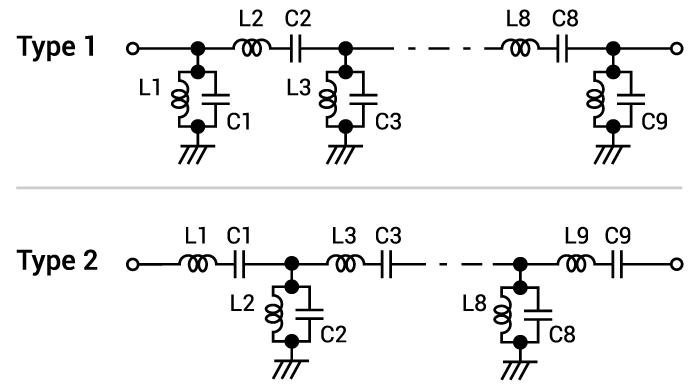# Bandpass Filter Calculator

## This calculates values of the inductance, capacitance of an inductor and capacitor used in a Butterworth LC bandpass filter.

(from 1 to 9)
MHz
MHz
Z
Z

Type 1
[nH]
[pF]

Type 2
[nH]
[pF]

### Calculating Inductance and Capacitance of a Bandpass Filter

This calculator helps determine the correct values of the inductance (L) and capacitance (C) of an inductor and capacitor to be used in a Butterworth LC bandpass filter. All that is needed is to input the desired cutoff frequency, the passband, the impedance, and the ripple. It is possible to have up to 9 stages of LC pair for this calculator.### Equations

$$L_{shunt_{i}} = \frac{g_{i}Z_{0}}{(2 \pi)^2 BW f_{c}}$$

$$L_{series_{i}} = \frac{BW f_{c} Z_{0}}{g_{i} (2 \pi f_{c})^2}$$

$$C_{shunt_{i}} = \frac{ BW }{g_{i} Z_{0} (2 \pi (f_{c})^2)}$$

$$C_{series_{i}} = \frac{g_{i}}{2 \pi BW Z_{0}}$$

Where:

$$n$$ = number of LC pair

$$i$$ = order of inductor or capacitor

$$f_{c}$$ = cutoff frequency

$$BW$$ = passband

$$r$$ = ripple in dB

$$rr = \frac{r}{17.37}$$

$$g_{i} = \frac{2 a_{i}}{g_{n}}$$  when $$i = 1$$

$$g_{i} = \frac{4 a_{i-1} a_{i}}{b_{i-1} g_{i-1}}$$

$$a_{i} = sin(\frac{(2i - 1) \pi}{2n})$$

$$b_{i} = g_{n}^2+sin(\frac{\pi i}{n})^2$$

$$g_{n} = \frac{e^{btn} - e^{-btn}}{2}$$

$$btn = \frac{log (\frac{e^{2rr}+1}{e^{2rr}-1})}{2^{n}}$$

### Applications of Butterworth Bandpass Filters

The Butterworth filter is a form of RF filter using lumped elements that is widely used in many radio frequency filter applications. The key feature of the Butterworth filter when compared to other forms of filters is that it has a nominally flat response within its pass-band and an adequate roll-off. As a result, the Butterworth filter may also be known as the maximally flat magnitude filter. The Butterworth filter is often considered as a good all-around form of filter adequate for many applications, although it does not provide the sharpest cut-off.

The key feature of the Butterworth filter is that it has a maximally flat response within the pass-band, i.e. it has no response ripples as in the case of many other forms of RF filters. There is a frequency known as the cut-off frequency which is is defined as the point on the Butterworth filter response where the power drops to half, i.e. the voltage drops to 71%, i.e. 1/√2 of its maximum amplitude at lower frequencies. It is also worth noting that the maximum amplitude, the minimum loss for the Butterworth filter response, occurs at 0 Hz or radians/s.

When plotted on logarithmic scales, the Butterworth filter response is flat within its pass-band and then rolls off with an ultimate linear roll off rate of -6 dB per octave (-20 dB per decade). A second-order filter decreases at -12 dB per octave, etc. The ultimate roll off rate is actually the same for all low pass and high pass filters.